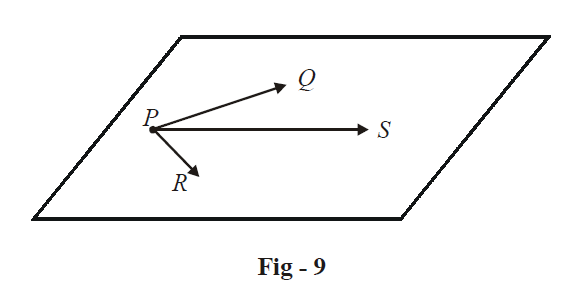# Examples on Equations of Three Dimensional Planes Set 1

Go back to  'Three Dimensional Geometry'

Example – 10

Find the equation of the plane passing through the points P(1, 1, 0), Q (1, 2, 1) and R (–2, 2, –1).

Solution: Let S(x, y, z) be any arbitrary point in the plane whose equation we wish to determine:Since  $$\overrightarrow{PQ}\times \overrightarrow{PR}$$  will be perpendicular to this plane, we must have

\begin{align}&\qquad\qquad\qquad \overrightarrow{PS}\cdot \left( \overrightarrow{PQ}\times \overrightarrow{PR} \right)=0 \\ & \Rightarrow \quad \left\{ \left( x-1 \right)\hat{i}+\left( y-1 \right)\hat{j}+z\hat{k} \right\}\cdot \left| \begin{matrix} {\hat{i}} & {\hat{j}} & {\hat{k}} \\ 0 & 1 & 1 \\ -3 & 1 & -1 \\\end{matrix} \right|=0 \\ & \Rightarrow \quad \left\{ \left( x-1 \right)\hat{i}+\left( y-1 \right)\hat{j}+z\hat{k} \right\}\cdot \left( -2\hat{i}-3\hat{j}+3\hat{k} \right)=0 \\ & \Rightarrow \quad 2\left( x-1 \right)+3\left( y-1 \right)-3z=0 \\ & \Rightarrow \quad 2x+3y-3z=5 \\ \end{align}

We could have proceeded alternatively as follows: using the result of the last example, any arbitrary plane through P(1, 1, 0) will be of the form

\begin{align}&\qquad\quad a\left( x-1 \right)+b\left( y-1 \right)+cz=0 \\ & \Rightarrow \quad {{\lambda }_{1}}\left( x-1 \right)+{{\lambda }_{2}}\left( y-1 \right)+z=0;{{\lambda }_{1}}=\frac{a}{c},\,{{\lambda }_{2}}=\frac{b}{c} \\ \end{align}

If this passes through Q(1, 2, 1) and R(–2, 2, –1), we have

${{\lambda }_{2}}+1=0$

and  $-3{{\lambda }_{1}}+{{\lambda }_{2}}-1=0$

$\Rightarrow {{\lambda }_{1}}=-\frac{2}{3},\,\,{{\lambda }_{2}}=-1$

Thus, the equation of the plane is

\begin{align} &\qquad -\frac{2}{3}\left( x-1 \right)-\left( y-1 \right)+z=0 \\\\ & \Rightarrow \quad 2\left( x-1 \right)+3\left( y-1 \right)-3z=0\\ \\ & \Rightarrow\quad 2x+3y-3z=5 \\ \end{align}

Example – 11

Find the equation of the plane intercepting lengths a, b and c on the x-, y- and z-axis respectively.

Solution: The plane passes through the points A(a, 0, 0), B (0, b, 0) and C(0, 0, c). For any variable point S (x, y, z) in this plane, we have (as discussed in the previous section),

\begin{align} &\qquad\qquad\quad \overrightarrow {AS} \cdot \left( {\overrightarrow {AB} \times \overrightarrow {AC} } \right) = 0 \\\\&\Rightarrow \quad \left\{ {\left( {x - a} \right)\hat i + y\hat j + z\hat k} \right\} \cdot \left| {\begin{array}{*{20}{c}} {\hat i}&{\hat j}&{\hat k} \\ { - a}&b&0 \\ { - a}&0&c \end{array}} \right| = 0\\ \\ &\Rightarrow \quad \left\{ {\left( {x - a} \right)\hat i + y\hat j + z\hat k} \right\} \cdot \left( {bc\hat i + ac\hat j + ab\hat k} \right) = 0 \\\\ &\Rightarrow \quad \left( {bc} \right)\left( {x - a} \right) + acy + abz = 0 \\\\ &\Rightarrow \quad bcx + acy + abz = abc \\ \\ &\Rightarrow\quad \boxed{\frac{x}{a} + \frac{y}{b} + \frac{z}{c} = 1} \\ \end{align}
This general equation has the same form as the equation of the line in intercept form; which further proves the analogy between the formulae in two and in three dimensions.

Learn from the best math teachers and top your exams

• Live one on one classroom and doubt clearing
• Practice worksheets in and after class for conceptual clarity
• Personalized curriculum to keep up with school